×#### Thank you for registering.

One of our academic counsellors will contact you within 1 working day.

Click to Chat

1800-1023-196

+91-120-4616500

CART 0

• 0

MY CART (5)

Use Coupon: CART20 and get 20% off on all online Study Material

ITEM
DETAILS
MRP
DISCOUNT
FINAL PRICE
Total Price: Rs.

There are no items in this cart.
Continue Shopping• Complete JEE Main/Advanced Course and Test Series
• OFFERED PRICE: Rs. 15,900
• View Details

```Chapter 1: Number System Exercise – 1.2

Question: 1

Express the following rational numbers as decimals:

(i) 42/100

(ii) 327/500

(iii) 15/4

Solution:

(i) By long division method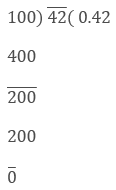Therefore, 42/100 = 0.42

(ii) By long division method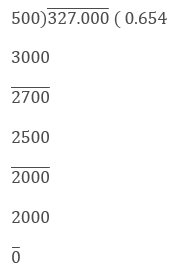Therefore, 327/500 = 0.654

(iii) By long division method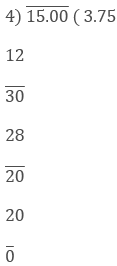Therefore, 15/4 = 3.75

Question: 2

Express the following rational numbers as decimals:

(i)  2/3

(ii) – (4/9)

(iii) – (2/15)

(iv) – (22/13)

(v)   437/999

Solution:

(i) By long division methodTherefore, 2/3 = 0.66

(ii) By long division method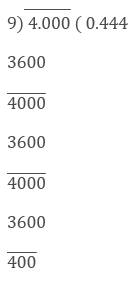Therefore, - 4/9 = - 0.444

(iii) By long division method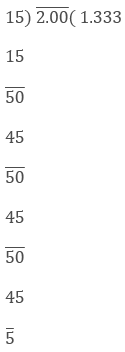Therefore, 2/15 = -1.333

(iv) By long division method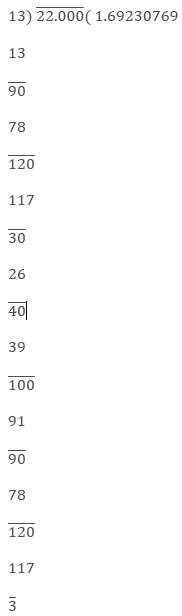Therefore, - 22/13 = - 1.69230769

(v) By long division methodTherefore, 437/999 = 0.43743

Question: 3

Look at several examples of rational numbers in the form of p/q (q ≠ 0), where p and q are integers with no common factor other than 1 and having terminating decimal representations. Can you guess what property q must satisfy?

Solution:

A rational number p/q is a terminating decimal

only, when prime factors of q are q and 5 only. Therefore,

p/q is a terminating decimal only, when prime

factorization of q must have only powers of 2 or 5 or both.
```### Course Features

• 728 Video Lectures
• Revision Notes
• Previous Year Papers
• Mind Map
• Study Planner
• NCERT Solutions
• Discussion Forum
• Test paper with Video Solution